# Modelling and Analysis of Hybrid PV-PEM Fuel Cell Power System

Modelling and Analysis of Hybrid PV-PEM Fuel Cell Power System
Electrical Engineering Department, Mohamed Boudiaf University, Msila 28000, Algeria

Mechatronics Laboratory (LMETR), Optics and Precision Mechanics Institute, Ferhat Abbas University, Setif 19000, Algeria

Corresponding Author Email:
a.harrag@univ-setif.dz
Page:
29-33
|
DOI:
https://doi.org/10.18280/psees.040104
14 May 2020
| |
Accepted:
12 November 2020
| | Citation

OPEN ACCESS

Abstract:

Among the renewable energy sources, photovoltaics seems to be the most promising source. It has the advantage of being available everywhere, friend of the environment and easily maintainable. Nevertheless, the major disadvantages are high manufacturing cost, low energy conversion efficiency and non-linear characteristics. On the other hand, the fuel cell is undoubtedly the least polluting means of making electrical energy. Indeed, apart from electricity, the reaction between hydrogen and air produces only water vapour. As such, the process does not reject any greenhouse gases, unlike the combustion of fossil fuels. Whether in a transport-type application (bus or car, or stationary, energy systems including PV or Fuel Cell systems have a in such systems, several levels of control are to be studied, modelled and optimized; this last point, according to one or more criteria of the whole system, can intervene at several levels: optimization of the topology as well as optimization of component sizing. This paper addresses the modelling of hybrid PV-PEM fuel cell power system where the whole system components are implemented using Matlab/Simulink environment. The main parts (PV and PEM fuel cell) are analyzed and investigated alone and in hybrid mode using different scenarios tests. The simulation results show the advantages of using such hybrid system in providing electrical energy in different use cases.

Keywords:

hybrid system, photovoltaic, fuel cell, PEMFC, MPPT, energy management

1. Introduction

In view of the increasing technological development around the world, which in turn leads to the increasing consumption of electric power, traditional energies no longer meet the needs required, it is necessary to find alternative energies to cover this deficit. On other hand be environmentally friendly due to the dangers resulting from pollution which affects the earth as a result of the use of these traditional energies. In order to overcome these problems, it has become necessary to move towards the use of alternative energies such as solar energy, wind, fuel cell, tidal, hydrogen etc. .

The development of new energy technologies should be mandatory. Among them, fuel cells and solar cells are promising energy technologies. Electricity generation using a hybrid energy system combining this both sources of energy is of great interest due to its several of field applications and industrial. Solar systems that convert sunlight into electricity, it has many advantages we mention are silent, low-maintenance, eco-friendly, clean and efficient independent of the size of generator .

Fuel cells are electro chemical (mechanical) devices that convert chemical energy into electricity without generating carbon dioxide (promising power generation with high efficiency and low environmental impact) . Among the many different fuel cell technologies, Proton Exchange Membrane (PEM) fuel cells are extensively used for mobile and portable applications. This is due to their compactness, low weight, high power density and clean, pollutant free operation. From the operational point of view, a relevant aspect is their low temperature of operation (typically 60-80℃), which allows fast starting times [4, 5].

This paper deals with the modelling of hybrid PV-PEM fuel cell power system where the whole system components are implemented using Matlab/Simulink environment. The main parts (PV and PEM fuel cell) are analyzed and investigated alone and in hybrid mode using different scenarios tests. The simulation results show the advantages of using such hybrid system in providing electrical energy in different use cases. The remainder of this paper is organized as follows. Section 2 presents the PV modelling; while the PEM fuel cell modelling is presented is described in Section 3. The simulation results and discussions are presented in Section 4. Finally, Section 5 draws the main conclusions of this work.

2. PV Modelling

The equivalent circuit of solar PV cell is shown in Figure 1, the cell consists of diode, a photo current and parallel/series resistor. The relation between the output current and voltage of PV module is given by :

$I=I_{p h}-I_{s}\left(e^{\frac{q\left(V+I R_{s}\right)}{N K T}}-1\right)-\frac{V+I R_{S}}{R_{s h}}$                        (1)

where, I is the output net current of PV cell; Iph is the light-generated photocurrent; Isc the short circuit current; Is the reverse saturation current of the diode; V is the cell output voltage; Rs the series resistor of the PV cell; Rsh the shunt resistor of the PV cell; q is the electron charge; A is the diode ideality factor; T is the working temperature of the p-n junction and K is the Boltzman’s constant.

## 1.jpg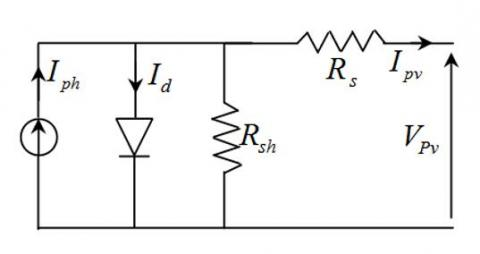Figure 1. Model of photovoltaic cell

The photo-current Iph is given by:

$I_{p h}=\left(I_{s c}+K_{i}\left(T-T_{r e f}\right)\right) \frac{G}{1000}$                        (2)

where, G is the sun irradiation; Ki the cell short circuit current temperature coefficient; and Tref is the nominal reference temperature.

The saturation current Is is described as follow:

$I_{s}=I_{\text {sref }}\left(\frac{T}{T_{r e f}}\right)^{3} e^{\left[\frac{1}{T_{r e f}}-\frac{1}{T}\right] \frac{q E_{g}}{N K T}}$                              (3)

where, Eg is the band gap energy of semiconductor; with:

$I_{\text {sref }}=\frac{I_{\text {scref }}}{\left(e^{\left[\frac{V_{\text {ocref }}}{K A T}\right]}-1\right)}$                           (4)

The output simulation results of the I-V and P-V curves for different values of irradiation and temperature are presented in Figures 2 and 3.

3. PEM Fuel Cell Modelling

Fuel Cell converts oxygen and hydrogen chemical energy to electrical energy during which heat and water is generated as well .

The half-reaction of oxidation of hydrogen will be as follows:

$\mathrm{H}_{2} \rightarrow 2 \mathrm{H}^{+}+2 \mathrm{e}^{-}$                          (5)

The half-reduction reduction of oxygen will be as follows:

$\mathrm{O}_{2}+4 \mathrm{e}^{-} \rightarrow 2 \mathrm{O}^{-2}$                        (6)

The overall reaction taking place at the fuel cell can be summarized as:

$\mathrm{H}_{2}+\frac{1}{2} \mathrm{O}_{2} \rightarrow \mathrm{H}_{2} \mathrm{O}+$ electricalenergy $+$ heat                     (7)

Each cell voltage can be defined by the well known expression given by:

$V_{F C}=E_{\text {nernst }}-V_{\text {act }}-V_{\text {ohmic }}-V_{\text {conc }}$                       (8)

Enernst is the reversible open circuit voltage approximated by [8-10]:

$\mathrm{E}_{\text {nernst }}=1.229-\left(8.5 \times 10^{-4}\right)(\mathrm{T}-298.15)+\left(4.385 \times 10^{-5} \mathrm{~T}\left[\ln \left(\mathrm{P}_{\mathrm{H} 2}\right)+0.5 \ln \left(\mathrm{P}_{\mathrm{O} 2}\right)\right]\right.$                            (9)

where, T is the temperature; PO2 the oxygen pressure and PH2 the hydrogen pressure.

## 2a.png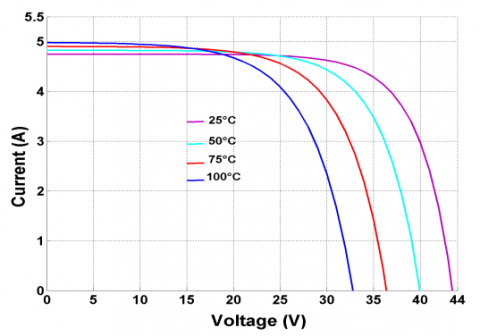(a)

## 2b.png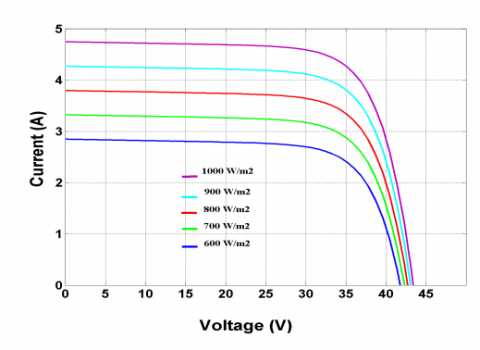(b)

Figure 2. I-V Characteristics: a) In case of temperature variation, b) In case of irradiation variation

## 3a.png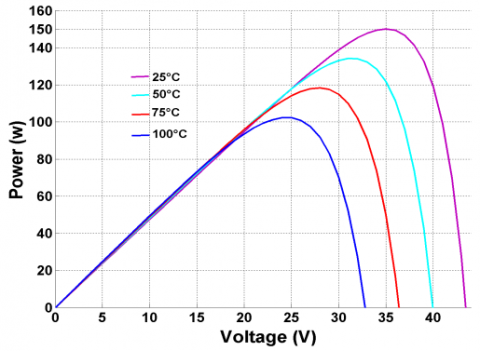(a)

## 3b.png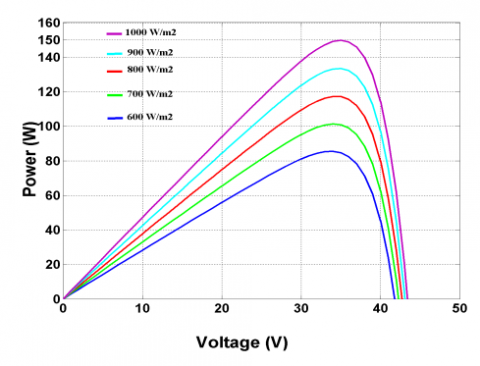(b)

Figure 3. PV Characteristics: a) In case of temperature variation, b) In case of irradiation variation

Vact is the activation voltage drop approximated by:

$V_{\mathrm{act}}=\xi_{1}+\xi_{2} \cdot \mathrm{T}+\xi_{3} \cdot \mathrm{T} \cdot \ln \left(\mathrm{C}_{\mathbf{0 2}}\right)+\xi_{4} \cdot \mathrm{T} \cdot \ln \left(\mathrm{i}_{\mathrm{FC}}\right)$                        (10)

where, $\xi_{\mathrm{i}}$ (i = 1 to 4) are parametric coefficients for each cell; iFC is the cell current and CO2 is the oxygen’s concentration.

Vohmic is the ohmic linear voltage drop proportional to electric current approximated by:

$\mathrm{V}_{\text {ohmic }}=R_{\text {ohmic }} \cdot i_{\mathrm{FC}}$                           (11)

where, iFC is the cell current; Rohmic is the sum of the contact resistance Rc and the membrane resistance Rm.

Vconc is the concentration voltage drop approximated by:

$\mathrm{V}_{\mathrm{conc}}=-\mathrm{b} \cdot \ln \left(1-\frac{\mathrm{i}_{\mathrm{FC}} / \mathrm{A}}{\mathrm{I}_{\max }}\right)$                             (12)

where, b is the concentration loss constant; iFC is the cell current; A is the is cell active area; Imax is the maximum current density.

Thus, the FC voltage and power given in Figure 4 are nonlinear functions of the FC current, internal temperature and pressure of oxygen and hydrogen gasses.

## 4a.png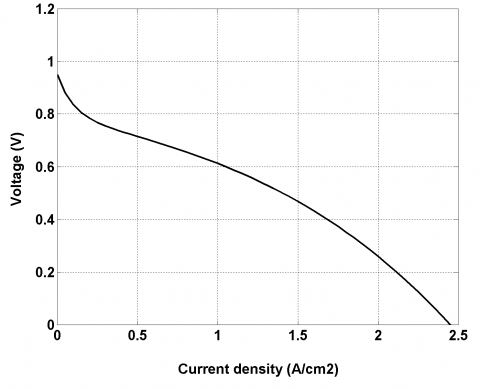(a)

## 4b.png(b)

Figure 4. FC Characteristic; a) V-I, b) P-I

4. Results and Discussion

Results of the modeling of photovoltaic system, when apply the two profile of irradiance and temperature during one day, are illustrated in Figures 5 and 6.

## 5.png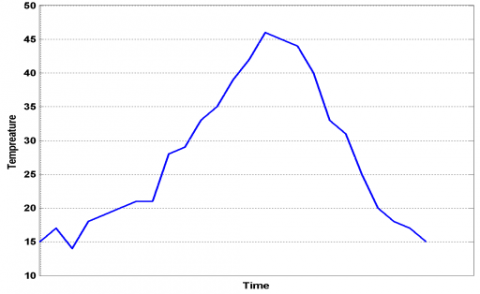Figure 5. Temperature variation

## 6.png## 7.png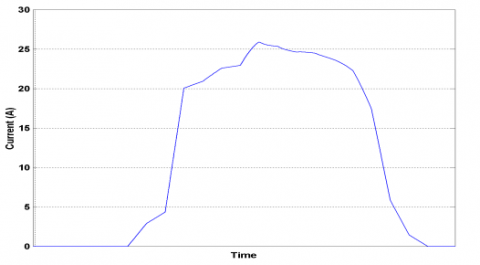Figure 7. Hybrid system current curve

## 8.pngFigure 8. Hybrid system voltage curve

## 9.png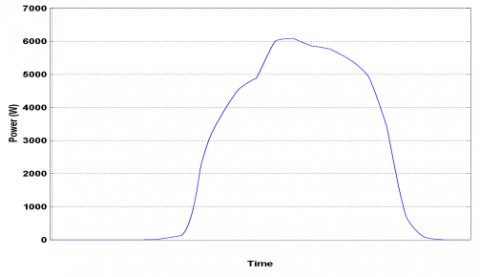Figure 9. Hybrid system power curve

Figures 7 to 9 show the generated current, voltage and power through the solar cell over one days. As shown, when the irradiance and temperature becomes higher or lower, the values changes accordingly.

5. Conclusion

This paper addresses the modeling of hybrid PV-PEM fuel cell power system where the whole system components are implemented using Matlab/Simulink environment. The main parts (PV and PEM fuel cell) are analyzed and investigated alone and in hybrid mode using different scenarios tests. The simulation results using different test scenarios including temperature and irradiation profile for one day show the advantages of using such hybrid system in providing electrical energy in different use cases.

Acknowledgment

The Algerian Ministry of Higher Education and Scientific Research via the DGRSDT supported this research (PRFU Project code: A01L07UN190120180005).

Nomenclature
 I The current of the PV cell (A). Iph The light-generated photocurrent (A). Is The reverse saturation current of the diode (A). Isc The Short circuit current (A). Q The electron charge (C). V The voltage of the PV cell U (V). Voc The Open-circuit voltage (V). Rs The series resistor of the PV cell (Ω). Rsh The shunt resistor of the PV cell (Ω). N The diode ideality factor. K The Boltzman’s constant. T The temperature (K). Ki The cell short circuit-current temperature coefficient. Tref The nominal reference temperature (K). S The irradiation (W/m2). Eg The band-gap energy of semiconductor ED The new step size; N The Scaling factor. $\upsilon_{r e v}$ The reversible voltage (V). $\upsilon_{a c t}$ The activation overvoltage (V). $\upsilon_{o h m}$ The ohmic overvoltage (V). $\Delta \mathrm{G}$ The Gibbs free energy change J/mol. F The faraday’s constant C/mol. Z The number of electron. $\eta_{\mathrm{F}}$ The Faraday efficiency (%). $\varphi_{\mathrm{H}_{2}}$ The total hydrogen production (mole/s). $\delta$ The area of electrode (m2). S The Coeff. for overvoltage on electrodes (V). Ki=1,2, 3 The Coeff. for overvoltage on electrodes. $\varepsilon_{1}$ The Parameters related to ohmic (Ω m2). $\varepsilon_{2}$ The resistance of electrolyte (Ω m2C). PV Photovoltaic. FC Fuel Cell. MPPT Maximum Power Point Tracking. MPP Maximum Power Point. PEM Proton Exchange Membrane Electrolyzer. DC Direct Current. P&O Perturbation and Observation. IC Incremental Conductance.
References

 Hatti, M. (2013). Optimization of fuzzy-logic controller for PV-PEM fuel cell hybrid system. Int Conf On control engineering & information Technology, 4: 23-28.

 Azizi, A., Pierre-Olivier, L., Omeiri, A., Amiar, A., Charki, A., Riou, O., Delaleux, F., Durastanti, J. (2018). Impact of the aging of a photovoltaic module on the performance of a gridconnected system. Solar Energy, 174: 445-454. https://doi.org/10.1016/j.solener.2018.09.022

 Harrag, A., Messalti, S. (2018). How fuzzy logic can improve PEM fuel cell MPPT performances? International of Hydrogen and Energy, 43(1): 537-550. https://doi.org/10.1016/j.ijhydene.2017.04.093

 Sankar, K., Jana, A.K. (2018). Nonlinear multivariable sliding mode control of a reversible PEM fuel cell integrated system. Energy Conversion and Management, 171: 541-565. https://doi.org/10.1016/j.enconman.2018.05.079

 Wang, T., Li, Q., Yin, L., Chen, W. (2019). Hydrogen consumption minimization method based on the online identification for multi-stack PEMFCs system. International Journal of Hydrogen Energy, 44(11): 5074-5081. https://doi.org/10.1016/j.ijhydene.2018.09.181

 Karami, N., Moubayed, N., Outbib, R. (2014). Energy management for a PEMFCPV hybrid system. Energy Conversion and Management, 82: 154-168. https://doi.org/10.1016/j.enconman.2014.02.070

 Senthil Kumar, A., Cermak, T., Misak, S., Horak, B. (2015). Modeling and simulation for 1.2kW PEM fuel cell power generation of isolated load conditions. International Conference on Electrical Drives and Power Electronics (EDPE), Tatranska Lomnica, Slovakia, pp. 235-240. https://doi.org/10.1109/EDPE.2015.7325299

 Mansour, A.M., Saad, N.H., Sattar, A.A. (2012). Maximum power point tracking of ten parameter fuel cell model. Journal of American Science, 8(8): 941-946.

 Dargahi, M., Rezanejad, M., Rouhi, J., Shakeri, M. (2009). Maximum power point tracking for fuel cell in fuel cell/battery hybrid systems. European Journal of Scientific Research, 25(4): 538-548. http://dx.doi.org/10.13140/RG.2.1.3770.7689

 Xu, L., Xiao, J. (2011). Modeling and simulation of PEM fuel cells based on electrochemical model. International Conference on Remote Sensing, Environment and Transportation Engineering, Nanjing, China, pp. 471-474. https://doi.org/10.1109/RSETE.2011.5964316